Function Repository Resource:

# CombinatorEncode

Encode an SK combinator expression into a list of 0s and 1s

Contributed by: Daniel Sanchez
 ResourceFunction["CombinatorEncode"][comb] encodes the combinator expression comb (composed of the symbols s and k) as a list of 0s and 1s. ResourceFunction["CombinatorEncode"][comb, fmt] yields the encoding of comb in the format fmt.

## Details and Options

The encoding (as a binary string)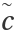of a combinator c is defined as: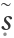00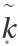01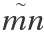1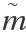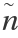ResourceFunction["CombinatorEncode"][{comb1, comb2, }] returns the encoding of each combinator.
Valid formats fmt include "Color", List, Number and String.
ResourceFunction["CombinatorEncode"][expr,{fmt1, fmt2, }] returns a list of differently formatted encoded expressions.
ResourceFunction["CombinatorEncode"] has the following option:
 CombinatorSymbols Automatic which combinator symbols should be used instead of s and k
The value of CombinatorSymbols should be an Association of the form <|"CombinatorS"s1,"CombinatorK"k1|>, where the symbols s1 and k1 will be encoded instead of s and k, respectively.

## Examples

### Basic Examples (2)

Encode a combinator expression into a unique list of 1s and 0s:

 In:=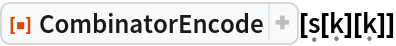Out=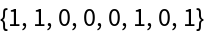Return the result of the encoding in various formats:

 In:=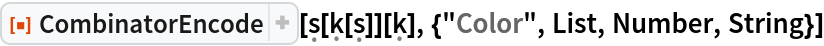Out=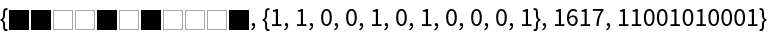### Options (1)

#### CombinatorSymbols (1)

Specify which combinator symbols to encode instead of s and k:

 In:=Out=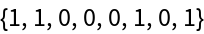### Applications (1)

Encode the five combinators that appear in Schönfinkel’s original paper:

 In:=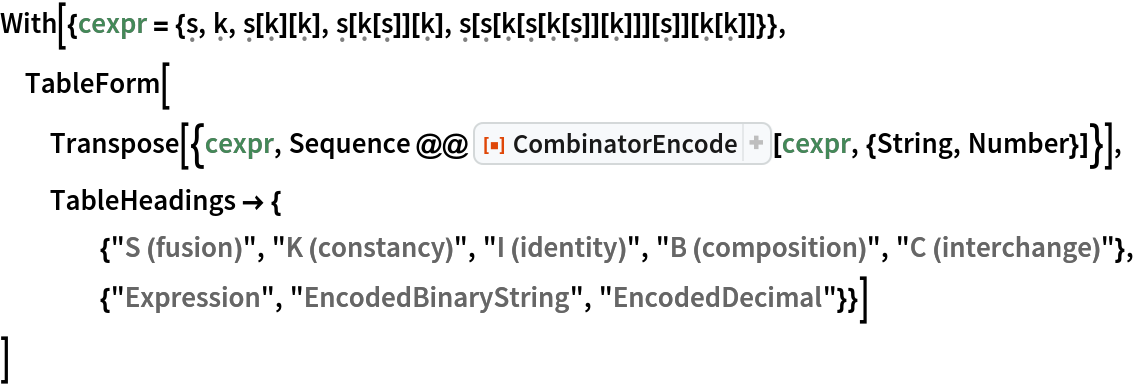Out=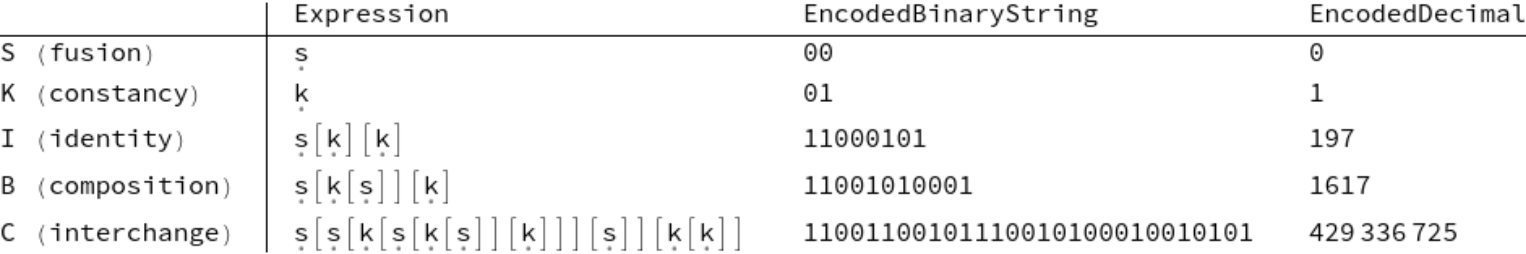### Properties and Relations (2)

Define a function for visualizing encodings:Visualize states of a multiway combinator evaluation graph:

 In:=Out=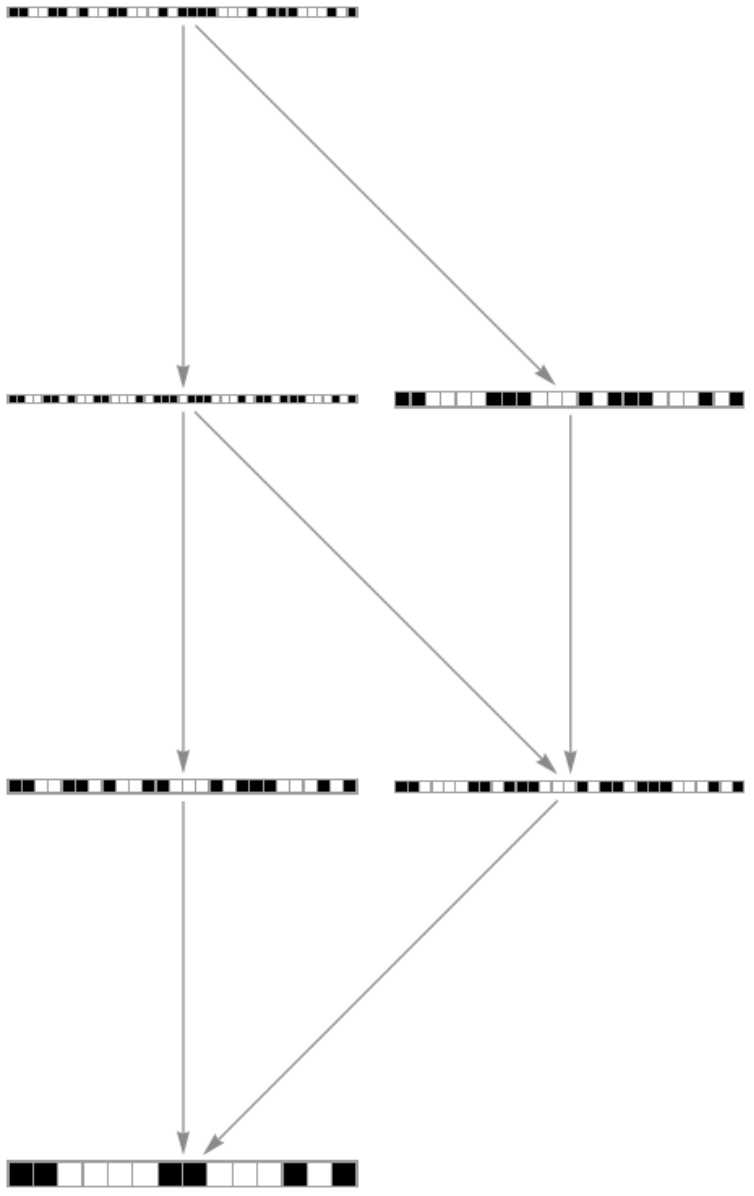### Neat Examples (2)

Plot the contraction of the Ω term, which reduces to itself after three steps:

 In:=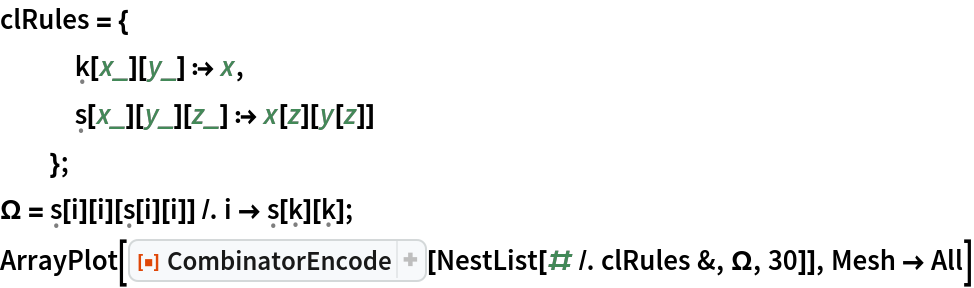Out=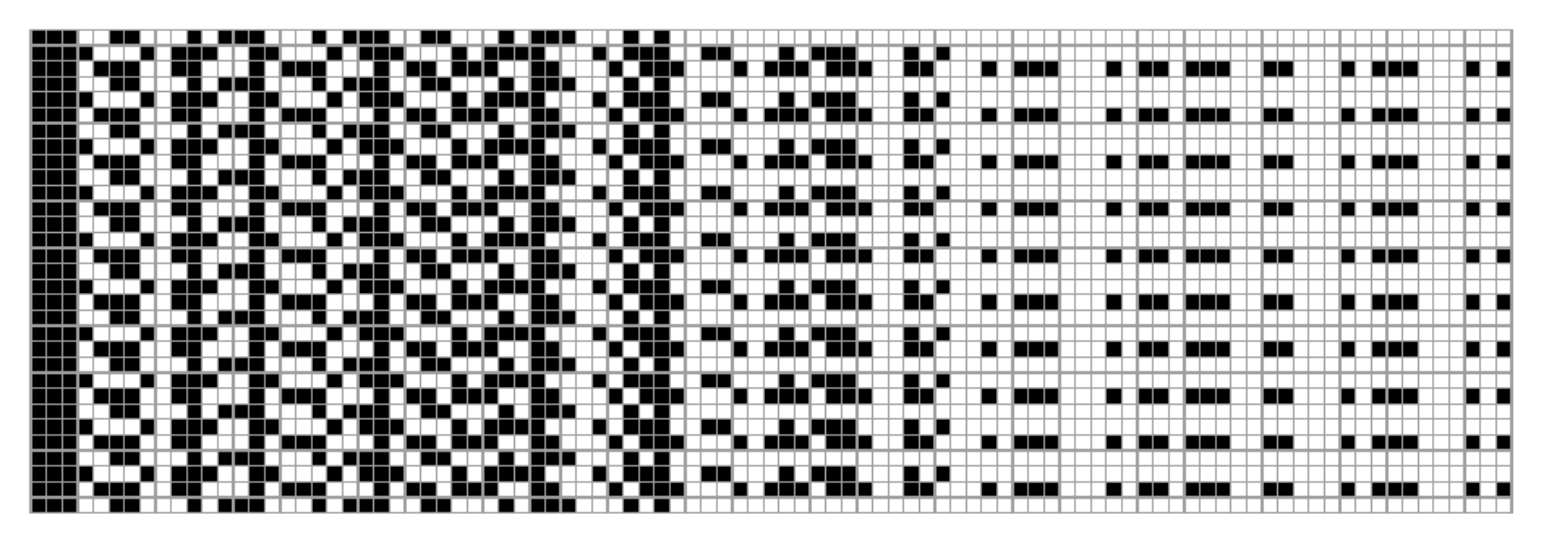Plot the successive applications of the successor function s[b] starting with k[i] (0):

 In:=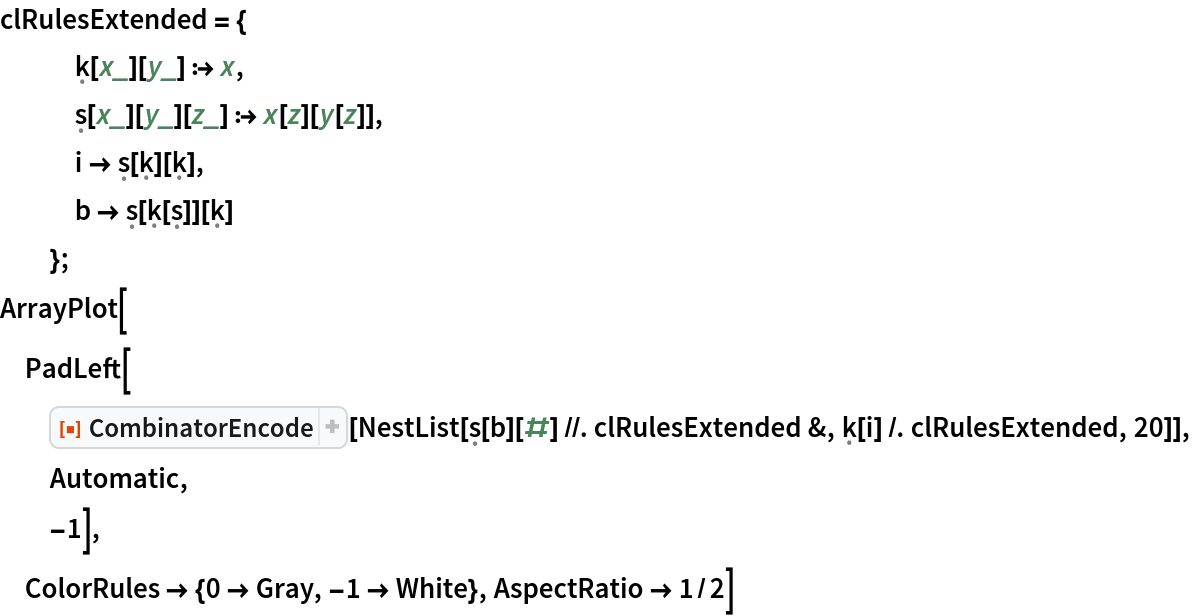Out=### Possible Issues (1)

Unknown symbols in the expression cannot be encoded:

 In:=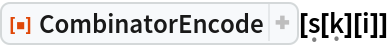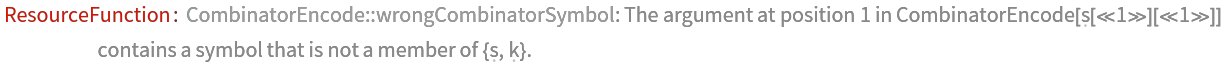Out=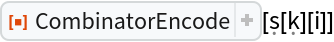In:=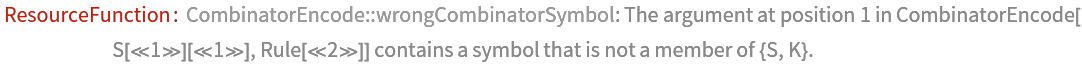Out=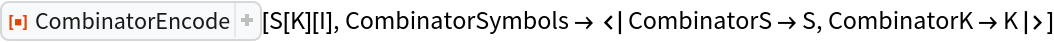Daniel Sanchez

## Version History

• 1.0.0 – 03 November 2020